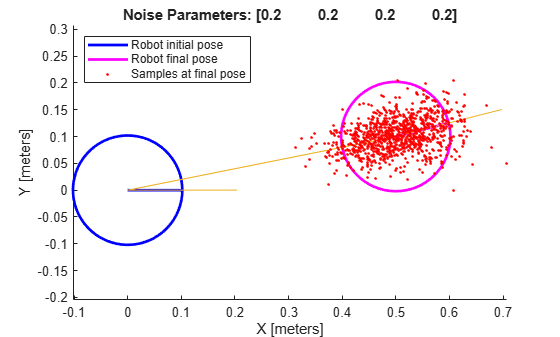# odometryMotionModel

Create an odometry motion model

Since R2019b

## Description

`odometryMotionModel` creates an odometry motion model object for differential drive vehicles. This object contains specific motion model parameters. You can use this object to specify the motion model parameters in the `monteCarloLocalization` object.

This motion model assumes that the vehicle makes pure rotation and translation motions to travel from one location to the other. The model propagates points for either forward or backwards motion based on these motion patterns. The elements of the `Noise` property refer to the variance in the motion. To see the effect of changing the noise parameters, use `showNoiseDistribution`.

## Creation

### Syntax

``omm = odometryMotionModel``

### Description

example

````omm = odometryMotionModel` creates an odometry motion model object for differential drive vehicles.```

## Properties

expand all

Gaussian noise for vehicle motion, specified as a 4-element vector. This property represents the variance parameters for Gaussian noise applied to vehicle motion. The elements of the vector correspond to the following errors in order:

• Rotational error due to rotational motion

• Rotational error due to translational motion

• Translational error due to translation motion

• Translational error due to rotational motion

Type of the odometry motion model, returned as `'DifferentialDrive'`. This read-only property indicates the type of odometry motion model being used by the object.

## Object Functions

 `showNoiseDistribution` Display noise parameter effects

## Examples

collapse all

This example shows how to use the `odometryMotionModel` class to predict the pose of a vehicle. An `odometryMotionModel` object contains the motion model parameters for a differential drive vehicle. Use the object to predict the pose of a vehicle based on its current and previous poses and the motion model parameters.

Create odometry motion model object.

`motionModel = odometryMotionModel;`

Define previous poses and the current odometry reading. Each pose prediction corresponds to a row in `previousPoses` vector.

```previousPoses = rand(10,3); currentOdom = [0.1 0.1 0.1];```

The first call to the object initializes values and returns the previous poses as the current poses.

`currentPoses = motionModel(previousPoses, currentOdom);`

Subsequent calls to the object with updated odometry poses returns the predicted poses based on the motion model.

```currentOdom = currentOdom + [0.1 0.1 0.05]; predPoses = motionModel(previousPoses, currentOdom);```

This example shows how to visualize the effect of different noise parameters on the `odometryMotionModel` class. An `odometryMotionModel` object contains the motion model noise parameters for a differential drive vehicle. Use `showNoiseDistribution` to visualize how changing these values affect the distribution of predicted poses.

Create a motion model object.

`motionModel = odometryMotionModel;`

Show the distribution of particles with the existing noise parameters. Each particle is a hypothesis for the predicted pose.

`showNoiseDistribution(motionModel);`Show the distribution with a specified odometry pose change and number of samples. The change in odometry is used as the final pose with hypotheses distributed around based on the `Noise` parameters.

```showNoiseDistribution(motionModel, ... 'OdometryPoseChange', [0.5 0.1 0.25], ... 'NumSamples', 1000);```Change the `Noise` parameters and visualize the effects. Use the same odometry pose change and number of samples.

``` motionModel.Noise = [0.2 1 0.2 1]; showNoiseDistribution(motionModel, ... 'OdometryPoseChange', [0.5 0.1 0.25], ... 'NumSamples', 1000);```## Limitations

If you make changes to your motion model after using it with the `monteCarloLocalization` object, call `release` on that object beforehand. For example:

```mcl = monteCarloLocalization; [isUpdated,pose,covariance] = mcl(ranges,angles); release(mcl) mcl.MotionModel.Noise = [0.25 0.25 0.4 0.4];```

 Thrun, Sebatian, Wolfram Burgard, and Dieter Fox. Probabilistic Robotics. MIT Press, 2005.